Study Guide

# Basic Algebra - Linear Relationships

## Linear Relationships

We've now worked pretty extensively with equations containing two variables. All the equations we've looked at so far have represented straight lines, so we call them linear equations. There are several different forms of linear equations, but they all have one thing in common: the variables don't have exponents (other than the invisible 1).

Ready for a newsflash? If any of the variables do have exponents, the equation isn't a straight line. It's a curve. Wild, right?

### Linear and Non-Linear Equations

For example: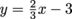is a linear equation because the variables all have an invisible exponent of 1 (which seems like they have no exponent). But the equation y = 3x2 + 1 is not a linear equation because the x variable has an exponent of 2. Here are a few more examples.

t = v – 5: linear equation
t = v2 – 5: non-linear equation

(y – 6) = 4(x + 1): linear equation
(y – 6) = 4(x + 1)3: non-linear equation

5x – 3y = 2x + 7: linear equation
5x(x) – 3y = 2x + 7: non-linear equation

### Proportional Linear Relationships

If a king-sized candy bar costs \$3.00 and we buy 1, we pay \$3.00 (just for giggles, we'll say there's no sales tax). If we buy 2 we pay \$6.00, if we buy 3 we pay \$9.00, and if we buy 4 we pay \$12.00. Let's put this info in a table so we can check it out a bit more. We'll say x is the number of candy bars purchased and y is the amount of money spent.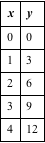They say imitation is the highest form of flattery, so the x variable in this relationship should be very flattered. Each time it increases, so does y, and by the same amount too. This is called a proportional relationship.

A picture is worth a thousand words, so let's graph it.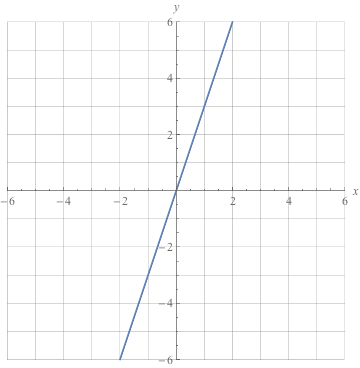Whaddaya know, proportional relationships are linear.

In this line, y goes up by 3 each time x goes up by 1, so the slope is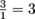(prove it by plugging two points into the slope formula). The line intersects the y-axis at 0, so the y-intercept is 0.

Plugging these into our slope-intercept formula, we can come up with an equation. Remember, m is the slope and b is the y-intercept.

y = mx + b
y = 3x + 0
y = 3x

The slope in this equation (3) is also called the constant of proportionality. Big word, small little number. In a nutshell, the constant of proportionality is the number we multiply x by to get y. Sometimes it's called a constant rate of change, too. So slope, constant of proportionality, and constant rate of change: all the same thing.

We can find the constant of proportionality from the table by dividing any y-value by its corresponding x-value (except 0, of course). We can find it from the graph by counting the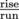between two points. We can find it in the equation by finding the slope, 'cause it's the same number.

A proportional relationship can be written as an equation.

y = kx

In this equation, y and x are variables and k is the constant of proportionality. Notice there's no y-intercept—they aren't allowed in proportional relationships. They muddle things up. In this type of equation, we say that y is directly proportional to x.

### Representations of a Linear Relationship

Linear relationships can be represented in several different ways: a graph, an equation, or a list of points. If we have one of these, with a little sleuth work we can figure out the other ones. We've already started with a linear equation and created a table of points and a graph. Rewind: if we start with a table of points or with a graph, we can go backwards and figure out the equation.
If we have a table of points that have a linear relationship, we can use two points to find the slope of the line. We can then graph the line to find the y-intercept, and use the slope and y-intercept to write an equation of the line.

### Sample Problem

Create a graph and write an equation for the linear relationship represented by the following points: (2, 5), (3, 6), (4, 7), (5, 8).

We're gonna need the slope, so let's start by tossing those first two points into our slope formula blender and making a delicious slope smoothie.Now we can graph the line and find the y-intercept.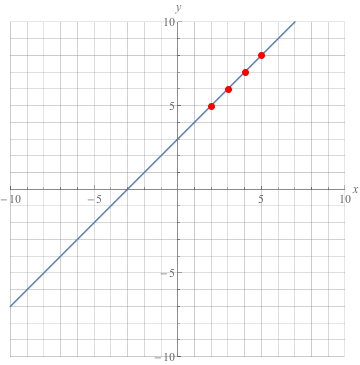The y-intercept is 3 and the slope is 1, so the equation of the line is y = x + 3.

### Forms of Linear Equations

Linear equations and inequalities are masters of disguise, and they can be written in lots of different forms. Don't let them fool you—they're still linear.

We can write an equation in standard form by moving the x and y terms to the same side of the equal sign. Standard form looks like this, where A, B, and C are real numbers.

Ax + By = C

The standard form of y = x + 3, for example, is yx = 3.

Another form of a linear equation that's super useful when we only know a few points is the point-slope form, which looks like this.

(yy1) = m(x – x1)

Click here to read more about the point-slope form of a linear equation.